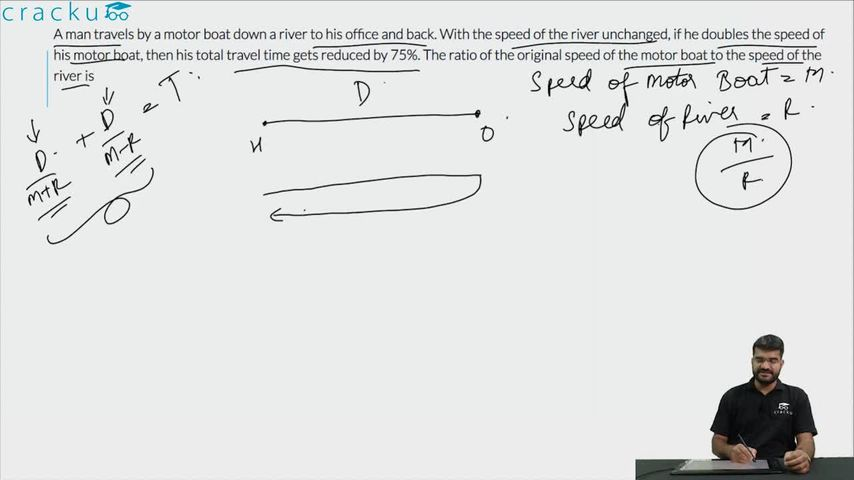Question 73

# A man travels by a motor boat down a river to his office and back. With the speed of the river unchanged, if he doubles the speed of his motor boat, then his total travel time gets reduced by 75%. The ratio of the original speed of the motor boat to the speed of the river is

Solution

Let the speed of the river be $$x$$ and the speed of the boat be $$u$$. Let $$d$$ be the one way distance and $$t$$ be the initial time taken.

Given,

$$t = \frac{d}{u - x} + \frac{d}{u + x}$$ ... i

Also,

$$\frac{t}{4} = \frac{d}{2u - x} + \frac{d}{2u + x}$$

$$t = \frac{4d}{2u - x} + \frac{4d}{2u + x}$$ ... ii

Equating both i and ii,

$$\dfrac{d}{u - x}$$+ $$\dfrac{d}{u + x}$$ =  $$\dfrac{4d}{2u - x} + \dfrac{4d}{2u + x}$$

$$\dfrac{2u}{u^2 - x^2} = \dfrac{16u}{4u^2 - x^2}$$

$$4u^2 - x^2 = 8u^2 - 8x^2$$

$$\frac{u^2}{x^2} = \frac{7}{4}$$

$$\frac{u}{x} = \frac{\sqrt{7}}{2}$$

### View Video Solution• All Quant CAT Formulas and shortcuts PDF
• 30+ CAT previous papers with solutions PDF